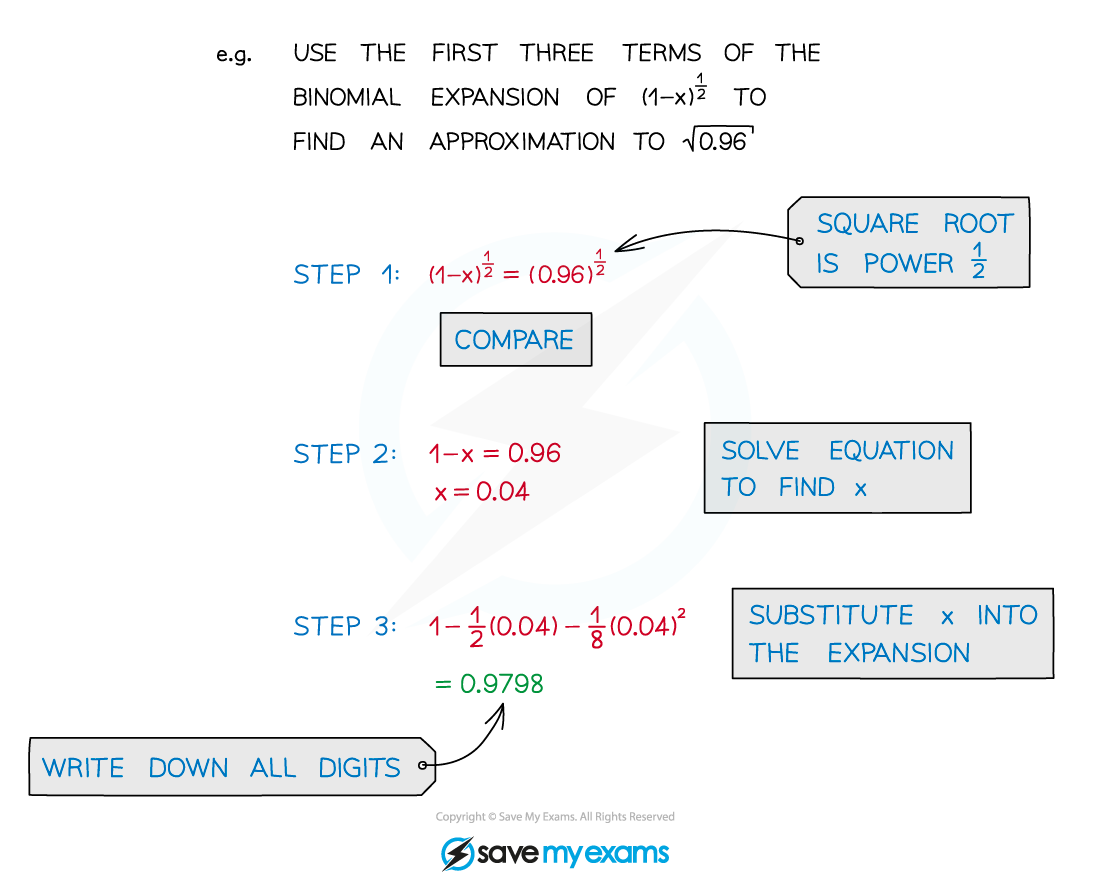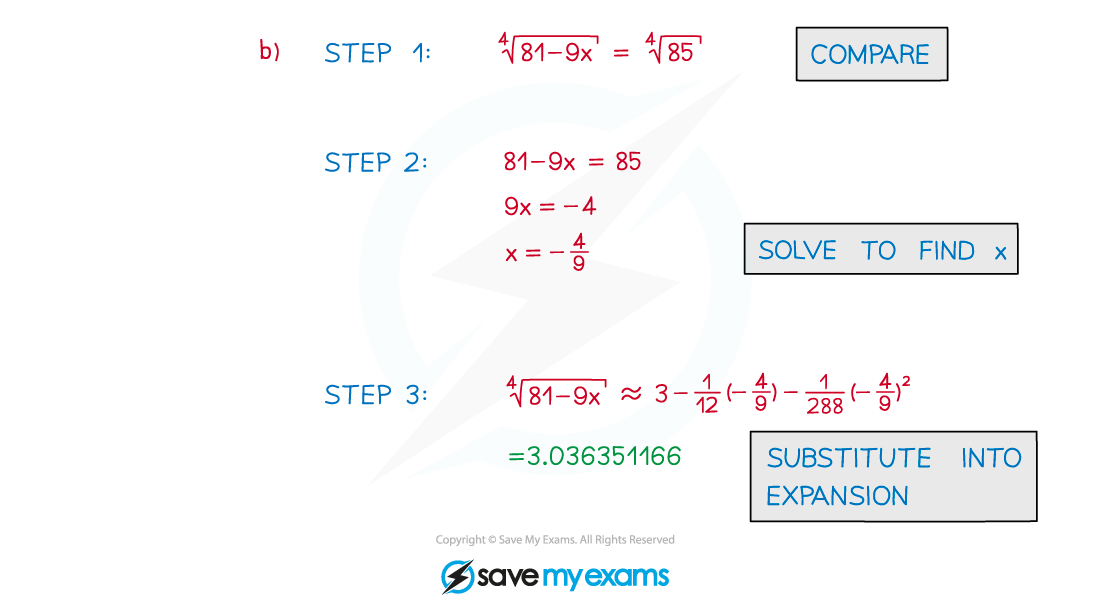# AQA A Level Maths: Pure复习笔记4.2.4 Approximating Values

### Approximating Values

#### What is the general binomial expansion?

• The general binomial expansion, as given in the formula booklet, is• If n ∈ ℕ then the expansion is finite (see Binomial Expansion)
• Otherwise the expansion is infinitely long
• It is only valid for |x| < 1 (-1 < x < 1)
• Only the first few terms of an expansion are usually needed

#### How do I use a binomial expansion to approximate a value?•  Ignoring higher powers of x leads to an approximation
• The more terms the closer the approximation is to the true value
• For most purposes, squared or cubed terms are accurate enoughSTEP 1  Compare the value you are approximating to (a + bx)n
STEP 2  Solve the appropriate equation to find the value of x
STEP 3  Substitute this value of x into the expansion to find the approximation

#### Exam Tip

• You can get a good idea if your approximation is correct by working out the “real” answer using your calculator.
• Sometimes it helps to factorise out a number before approximating

#### Worked Example